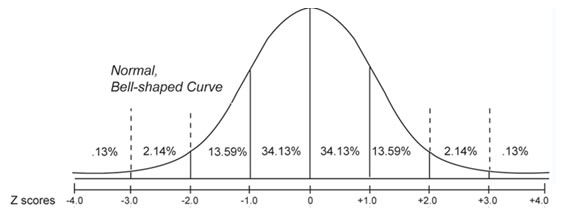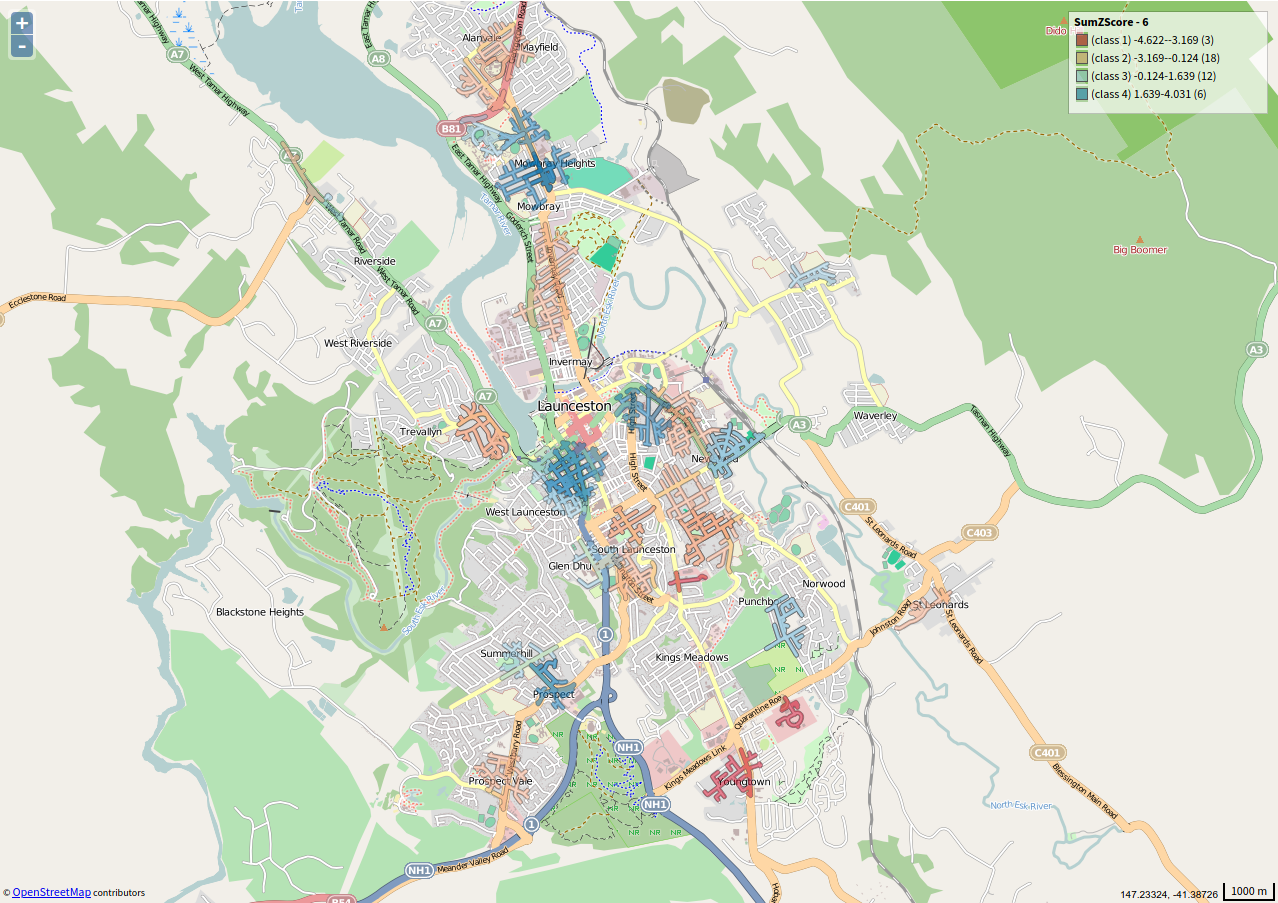# Walkability Index Within Areas

The Walkability Index Within Areas tool combines the three composite elements of the built urban form – Calculate Connectivity, Calculate Land Use Mix, and Calculate Gross Density to provide you with a comprehensive walkability index for your neighbourhoods in a single workflow.

Note: The walkability tools work optimally on regions around the size of local government areas. Study areas larger than this may be faced with long processing times.

### SET UP

This tutorial relies on you having first completed the workflow outlined in the Catchment Generator tool, as the outputs of that workflow are required to complete this use case.

When you have completed that tool, you will need to add one more additional dataset to drive this Walkability Index Within Areas workflow:

• Select Launceston SA3 (Australia →Tasmania → Rest of Tasmania → Launceston and North East → Launceston) as your area if it isn’t already setup from the Catchment Generator tool.
• Select ABS – Usual Residential Population and Dwelling Count (MB) 2016 with the following attributes:

• Geometry
• Mesh Block Category
• Total Usual Residential Population 2011

We add these as we need both land use data and population data to generate the scores for our neighbourhoods.

### Inputs

We are now ready to generate the output datasets. Open the tool (Tools → Walkability → Walkability Index Within Areas) and enter your parameters as shown below. These are also explained under the image.

• Road Network dataset: Line data set representing a road or pedestrian network. Select OpenStreetMap – Lines (Australia) 2017 in this instance.
• Areas:  This is where you specify the catchments that will have their walkability ranked. Select the catchments that you created with the Catchment Generator tool.
• Land use polygon dataset: This is the dataset that we use to specify the different land uses to be included in the Land Use Mix component of the walkability index. Select ABS – Usual Residential Population and Dwelling Count (MB) 2016.
• Land use classification attribute: This is where specify the attribute that has the different land uses within it. Select Mesh Block Category.
• Land use classifications: Classification categories values that you would like to include within your land use mix calculations. Select all values here, however, you might avoid ‘water’ or other land uses you are not interested in walking from/to.
• Population dataset: The dataset that has the population counts for your regions. Select ABS – Usual Residential Population and Dwelling Count (MB) 2016 dataset has population counts for each mesh block, so we select that.
• Population attribute: The attribute within your population dataset that contains the population counts – Select Total Usual Residential Population 2016.

Once you have entered your parameters, click Run Tool to execute the tool.### Outputs

Once your tool has run, click on the Display button to bring up the output of the tool. This is a table, with a large amount of information for about each of the catchments around the child care centres in the analysis (shown below). These are explained in some detail under the image.• Connectivity: The total number of connections per square kilometre.
• Area: The total area in square metres of each walking catchment.
• Connections The total number of connections in each of the walking catchment.
• LUM_X: The total square metres of each land use falling within each walking catchment.
• LandUseMixMeasure: This is an ‘entropy measure’, measuring the extent to which there is an equal distribution of each land use within the catchments. It is calculated by:

$\LARGE{{\sum_{l = 1}^{l} \left(P_{li}\right)\cdot ln\left(1/{P_{li}}\right)}\over{ln\left(n\right)}}$

Where $P_{li}$ is the proportion that each landuse $l$ contributes to each catchment $i$ and where $n$ represents the total number of landuse categories available. Values of the land use mix range form 0 (the lowest mix) to 1 (the highest possible mix).

• AverageDensity: The average population per hectare for each of the catchments. This is the Gross Density rather than Net Density.
• XXX_ZScore: These are the scores for the three different components (connectivity, land use and average density) converted into Z scores, where the mean for the different catchments is zero, and the numbers indicate how many standard deviations each score is above or below the mean. Essentially, the more positive the number, the better the relative score for that attribute, and the more negative number, the worse the relative score for that attribute. This is represented in the image below, and is calculated by the formula:

$\LARGE{Z_{i} = {X_{i} - \overline{X}}\over{s}}$

Where $X_i$ is the individual score for observation $i$, $\overline{x}$ is the mean of all the scores, and $s$ represents the sample standard deviation.We recommend that you make sure you have a relatively large number of observations (a minimum of 30) before using Z scores in any discussion, as they rely on robust mean and standard deviation calculations, which are less reliable at smaller samples sizes.

• SumZScore: This is the final Walkability Index for your catchments – and represents the sums of each of the different component Z score.

### VISUALISATION

We will now take a look at the distribution of the Walkability Index across our study areas. To do this, create a Choropleth of the Sum Z Score, choosing a Diverging palette type so that the middle colour represents the mean values. It should look something like the image below. We can see that the better walkability scores (blue) tend to be found in the north around Mowbray Heights and in central Launceston. If you hover over each of the catchments, you can see its individual attributes, and determine which of the different components let down or improved its overall walkability index.### Looking for Spatial Data?

You can browse the AURIN Data Discovery:### How can you Create Impact?